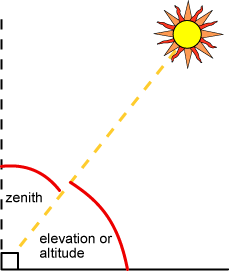# 仰角

v仰角（可与高度角互换使用）是太阳到水平线的角高度。令人困惑的是，高度和海拔也都用来描述海平面上的高度，以米为单位。在日出时，仰角为0°，当太阳直接在头顶上方时，仰角为90°（例如，在春分和秋分的赤道处）。

$\alpha =90+\phi -\delta$

φ 是我们该位置的纬度（北半球为正值，南半球为负值），
δ 是太阳偏角，取决于一年当中的第几天。

## Elevation Angle

$\alpha ={\mathrm{sin}}^{-1}\left[\mathrm{sin}\delta \mathrm{sin}\phi +\mathrm{cos}\delta \mathrm{cos}\phi \mathrm{cos}\left(HRA\right)\right]$

## Zenith Angle

$\zeta ={90}^{0}-\alpha$## Sunrise Time

$Sunrise=12-\frac{1}{{15}^{0}}{\mathrm{cos}}^{-1}\left(\frac{-\mathrm{sin}\phi \mathrm{sin}\delta }{\mathrm{cos}\phi \mathrm{cos}\delta }\right)-\frac{TC}{60}$

## Sunset Time

$Sunset=12+\frac{1}{{15}^{0}}{\mathrm{cos}}^{-1}\left(\frac{-\mathrm{sin}\phi \mathrm{sin}\delta }{\mathrm{cos}\phi \mathrm{cos}\delta }\right)-\frac{TC}{60}$

## Sunrise Time (Simplified)

$Sunrise=12-\frac{1}{{15}^{0}}{\mathrm{cos}}^{-1}\left(-\mathrm{tan}\phi \mathrm{tan}\delta \right)-\frac{TC}{60}$

## Sunset Time (simplified)

$Sunset=12+\frac{1}{{15}^{0}}{\mathrm{cos}}^{-1}\left(-\mathrm{tan}\phi \mathrm{tan}\delta \right)-\frac{TC}{60}$